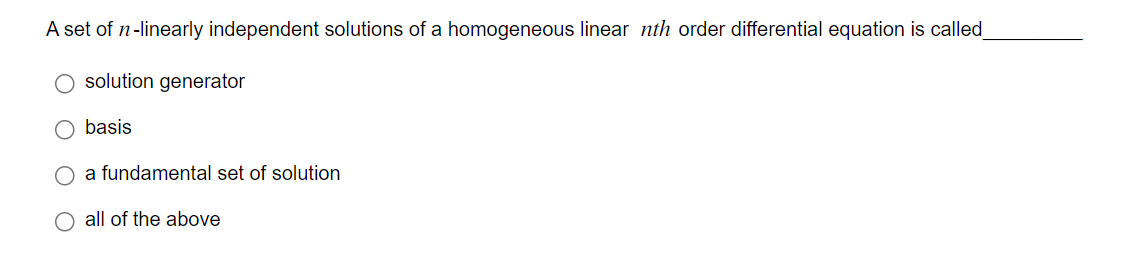# Question A set of $$n$$-linearly independent solutions of a homogeneous linear $$n t h$$ order differential equation is called solution generator basis a fundamental set of solution all of the aboveTranscribed Image Text: A set of $$n$$-linearly independent solutions of a homogeneous linear $$n t h$$ order differential equation is called solution generator basis a fundamental set of solution all of the above
Transcribed Image Text: A set of $$n$$-linearly independent solutions of a homogeneous linear $$n t h$$ order differential equation is called solution generator basis a fundamental set of solution all of the above&#12304;General guidance&#12305;The answer provided below has been developed in a clear step by step manner.Step1/2Solutions of linear Homogenous Equations : ExplanationDefinition of Fundamental Set of Solution : A set of n linearly independent non-trivial solution of nth order linear homogenous equation is called Fundamental set of solution.So, As given the set of solution is fundamental set of solution.Also, If we multiply fundamental set of solution by any constant , then the resulting set will be also solution of the homogenous $$\mathrm{\text{ODE}}$$.He ... See the full answer